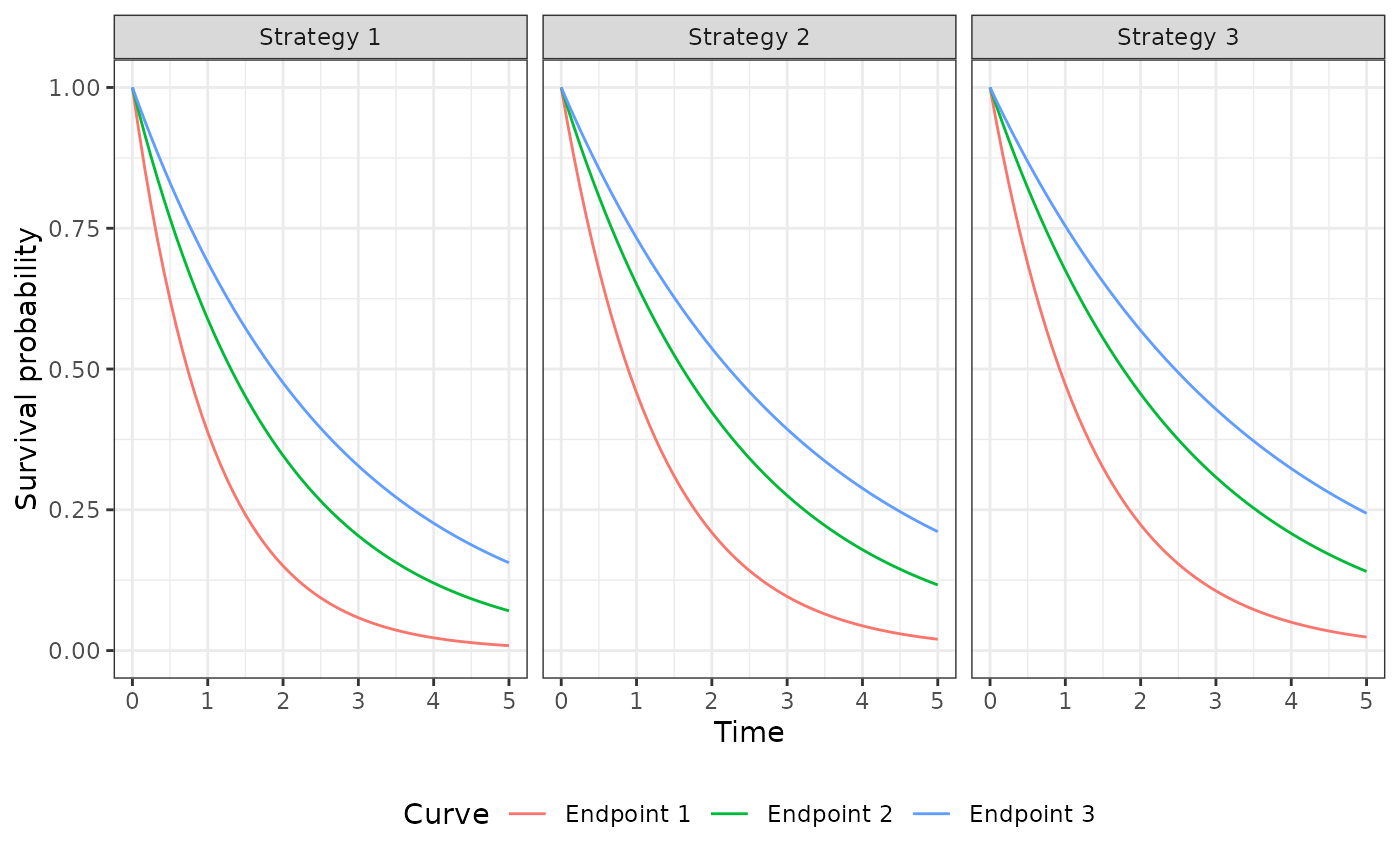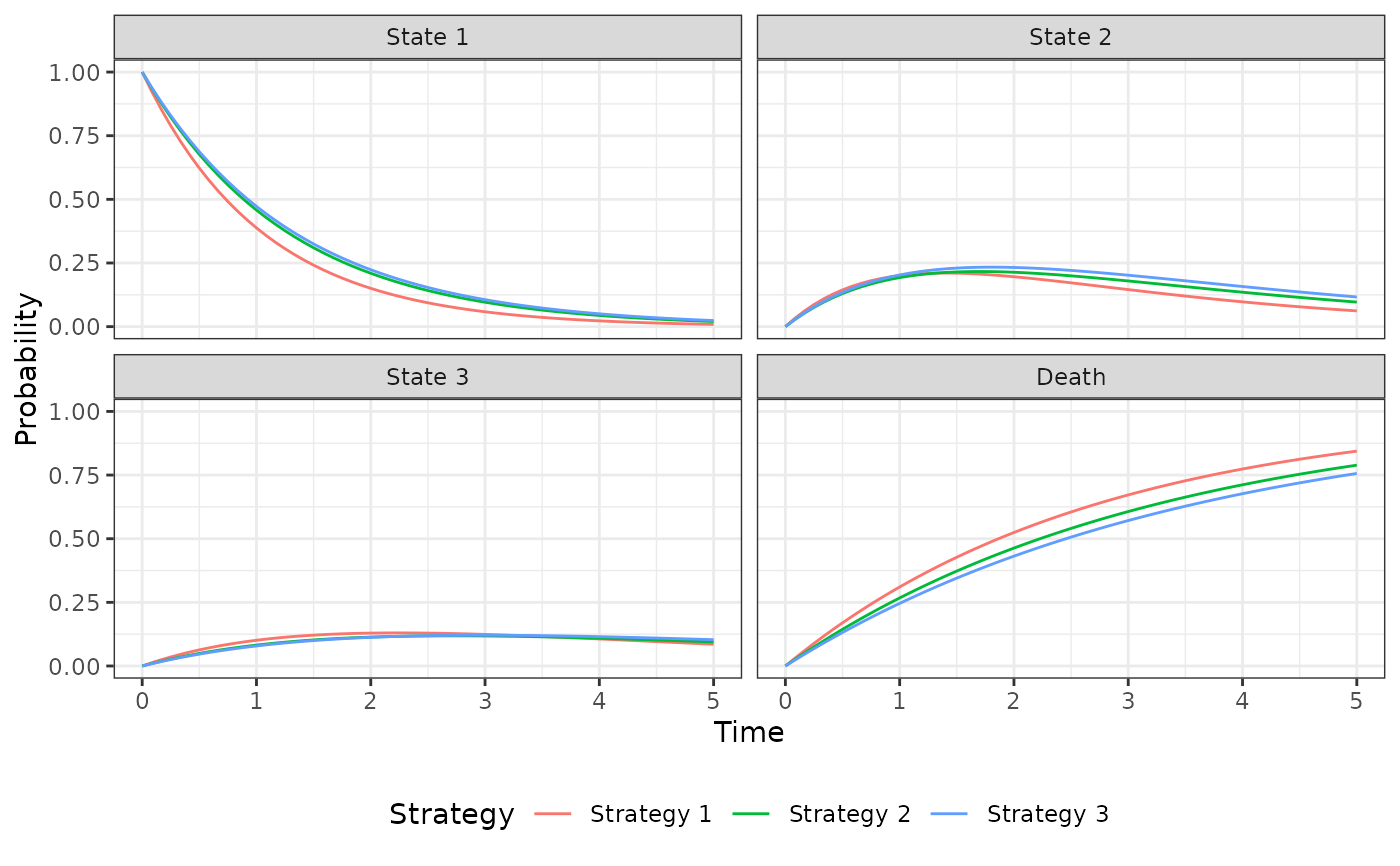Simulate outcomes from an N-state partitioned survival model.

Format

An R6::R6Class object.

Incerti and Jansen (2021). See Section 2.3 for a mathematical description of a PSM and Section 4.2 for an example in oncology. The mathematical approach used to simulate costs and QALYs from state probabilities is described in Section 2.1.

The PsmCurves documentation describes the class for the survival models and the StateVals documentation describes the class for the cost and utility models. A PsmCurves object is typically created using create_PsmCurves(). The PsmCurves documentation provides an example in which the model is parameterized from parameter objects (i.e., without having the patient-level data required to fit a model with R). A longer example is provided in vignette("psm").

Public fields

survival_models

The survival models used to predict survival curves. Must be an object of class PsmCurves.

utility_model

The model for health state utility. Must be an object of class StateVals.

cost_models

The models used to predict costs by health state. Must be a list of objects of class StateVals, where each element of the list represents a different cost category.

n_states

Number of states in the partitioned survival model.

t_

A numeric vector of times at which survival curves were predicted. Determined by the argument t in $sim_curves(). survival_ An object of class survival simulated using sim_survival(). stateprobs_ An object of class stateprobs simulated using$sim_stateprobs().

qalys_

An object of class qalys simulated using $sim_qalys(). costs_ An object of class costs simulated using$sim_costs().

Methods

Method new()

Create a new Psm object.

Method sim_stateprobs()

Simulate health state probabilities from survival_ using a partitioned survival analysis.

Psm$sim_stateprobs() Returns An instance of self with simulated output of class stateprobs stored in stateprobs_. Method sim_qalys() Simulate quality-adjusted life-years (QALYs) as a function of stateprobs_ and utility_model. See sim_qalys() for details. Usage Psm$sim_qalys(
dr = 0.03,
integrate_method = c("trapz", "riemann_left", "riemann_right"),
lys = TRUE
)

Arguments

dr

Discount rate.

integrate_method

Method used to integrate state values when computing costs or QALYs. Options are trapz for the trapezoid rule, riemann_left for a left Riemann sum, and riemann_right for a right Riemann sum.

lys

If TRUE, then life-years are simulated in addition to QALYs.

Returns

An instance of self with simulated output of class qalys stored in qalys_.

Method sim_costs()

Simulate costs as a function of stateprobs_ and cost_models. See sim_costs() for details.

Arguments

by_grp

If TRUE, then costs and QALYs are computed by subgroup. If FALSE, then costs and QALYs are aggregated across all patients (and subgroups).

Method clone()

The objects of this class are cloneable with this method.

Psm$clone(deep = FALSE) Arguments deep Whether to make a deep clone. Examples library("flexsurv") library("ggplot2") theme_set(theme_bw()) # Model setup strategies <- data.frame(strategy_id = c(1, 2, 3), strategy_name = paste0("Strategy ", 1:3)) patients <- data.frame(patient_id = seq(1, 3), age = c(45, 50, 60), female = c(0, 0, 1)) states <- data.frame(state_id = seq(1, 3), state_name = paste0("State ", seq(1, 3))) hesim_dat <- hesim_data(strategies = strategies, patients = patients, states = states) labs <- c( get_labels(hesim_dat), list(curve = c("Endpoint 1" = 1, "Endpoint 2" = 2, "Endpoint 3" = 3)) ) n_samples <- 2 # Survival models surv_est_data <- psm4_exdata$survival fit1 <- flexsurvreg(Surv(endpoint1_time, endpoint1_status) ~ factor(strategy_id), data = surv_est_data, dist = "exp") fit2 <- flexsurvreg(Surv(endpoint2_time, endpoint2_status) ~ factor(strategy_id), data = surv_est_data, dist = "exp") fit3 <- flexsurvreg(Surv(endpoint3_time, endpoint3_status) ~ factor(strategy_id), data = surv_est_data, dist = "exp") fits <- flexsurvreg_list(fit1, fit2, fit3) surv_input_data <- expand(hesim_dat, by = c("strategies", "patients")) psm_curves <- create_PsmCurves(fits, input_data = surv_input_data, uncertainty = "bootstrap", est_data = surv_est_data, n = n_samples) # Cost model(s) cost_input_data <- expand(hesim_dat, by = c("strategies", "patients", "states")) fit_costs_medical <- lm(costs ~ female + state_name, data = psm4_exdata$costs$medical) psm_costs_medical <- create_StateVals(fit_costs_medical, input_data = cost_input_data, n = n_samples) # Utility model utility_tbl <- stateval_tbl(tbl = data.frame(state_id = states$state_id, min = psm4_exdata$utility$lower, max = psm4_exdata$utility$upper), dist = "unif") psm_utility <- create_StateVals(utility_tbl, n = n_samples, hesim_data = hesim_dat) # Partitioned survival decision model psm <- Psm$new(survival_models = psm_curves, utility_model = psm_utility, cost_models = list(medical = psm_costs_medical)) psm$sim_survival(t = seq(0, 5, 1/12)) autoplot(psm$survival_, labels = labs, ci = FALSE, ci_style = "ribbon")psm$sim_stateprobs() autoplot(psm$stateprobs_, labels = labs)psm$sim_costs(dr = .03) head(psm$costs_)
#> sample strategy_id patient_id grp_id state_id dr category costs #> 1: 1 1 1 1 1 0.03 medical 32040.82 #> 2: 1 1 1 1 2 0.03 medical 17241.33 #> 3: 1 1 1 1 3 0.03 medical 17165.95 #> 4: 1 1 2 1 1 0.03 medical 32040.82 #> 5: 1 1 2 1 2 0.03 medical 17241.33 #> 6: 1 1 2 1 3 0.03 medical 17165.95
head(psm$sim_qalys(dr = .03)$qalys_)
#> sample strategy_id patient_id grp_id state_id dr qalys lys #> 1: 1 1 1 1 1 0.03 0.8759927 1.0170828 #> 2: 1 1 1 1 2 0.03 0.4895583 0.6482033 #> 3: 1 1 1 1 3 0.03 0.3468770 0.5055821 #> 4: 1 1 2 1 1 0.03 0.8759927 1.0170828 #> 5: 1 1 2 1 2 0.03 0.4895583 0.6482033 #> 6: 1 1 2 1 3 0.03 0.3468770 0.5055821